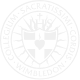# Mathematics

### A solid grounding in Mathematics is a prerequisite for the study of many other subjects, particularly the sciences.  It is also a sought after skill by employers due to its emphasis on problem solving and its reputation as a particularly rigorous discipline.

#### Lower Line

The programme of study for key stage 3 is organized into distinct domains, but pupils should build on key stage 2 and make connections across mathematical ideas to develop fluency, mathematical reasoning and competence in solving increasing sophisticated problems.

Fluency will be developed by consolidating what has been learnt at key stage 2 and extending understanding to include decimals, fractions, powers and roots, by using algebra to generalise the structure of arithmetic and to formulate mathematical relationships.  There will also be an emphasis on geometry, statistical probability, interpreting and representing data, and elementary trigonometry.

#### Figures Term 1

• Sequences and Functions Resource
• Decimals and Negative Numbers
• Length, Perimeter and Area
• Order of Operations

#### Figures Term 2

• Fractions, Decimals and Percentages
• Working with Data
• Chance and Probability
• Formulae, Functions and Equations
•  Surveys, Experiments and Probability

#### Figures Term 3

• Mental and Written Methods
• Using a Calculator
• Expressions, Equations and Functions
• Measures, Triangles and 3D Shapes
• Fractions, Percentages and Ratios
• Functions and Graphs

#### Rudiments Term 1

• Powers, Multiples, Factors and Primes
• Generating and Describing Sequences
• Angles, Lines and Shapes
• Chance and Probability  Fractions and Decimals

#### Rudiments Term 2

• Percentages
• Simplifying
• Expressions
• Equations and
• Formulae
• Equations and
• Formulae
• Functions

#### Rudiments Term 3

• Place value and rounding
• Mental Methods and using a calculator
• Transformations
• Surveys and analysing data
• Representing and Interpreting Data
• Order of operations and Checking

#### Lower Grammar 1

• Algebra
• Area and volume
• Statistics
• ​Fractions

#### Lower Grammar 2

• Reflections and enlargements
• Scale Drawings
• More Algebra- trial and improvement
• Fractions and
• Percentages

#### Lower Grammar 3

• Construction
• Loci
• 3 D Shapes
• Further Statistics
• Graphs and real life graphs
• Pythagoras
• Prisms and Cylinders

#### Higher Line

Maths in Year 10 will develop the concepts used at KS3. The main focus in the first term will be the Statistics element that is required for the GCSE examination. Then the course will further develop algebraic manipulation and trigonometry. All groups will start the higher linear course except the two Set A groups who will sit the GCSE in Statistics at the end of year 10 and then complete the GCSE in Maths at the end of year 11. This means that the top set will gain two mathematics qualifications by the end of their Syntax year which will stand them in good stead for further studies in this subject.

GSCE Mathematics will cover in more depth topics introduced at key stage 3.  Algebra will move beyond basic manipulation to methods of quadratic factorisation, such as completing the square.  The study of data handling and representation will continue to include cumulitive frequency and distributions.  Trigonometry and basic vectors will also be major topics.

There will also be a programme of assessments that will determine possible set changes.

#### Grammar Term 1

•   Number
•   Statistics
•   Angles
•   Fractions
•   Sequences
•   Measure

#### Grammar Term 2

•   Algebra
•   2 D Shapes
•   Equations
•   Percentages

#### Grammar Term 3

•  Graphs
•  More algebra
•  Inequalities

#### Syntax Term 1

• Estimating Accuracy
• Formulae
• Pythagoras' Theorem and Trigonometry 1
• Ratio and Proportion
• Three-Dimensional
• Shapes
• Graphs 2

#### Syntax Term 2

• Probability
• Further Graphs: Trial and Improvement
• Indices, Standard Form and Surds
• Constructions, Loci and Congruence
• Further Factorising,
• Simplifying,
• Completing the Square and Algebraic Proof

#### Syntax Term 3

• Circle Geometry
• Vectors
• Statistics
• Transformation of graphs

#### In Sixth Form, A levels in Mathematics and Further Mathematics are offered, with the latter option designed to stretch the most able students.

A level Maths involves the study of pure maths (such as algebra and surds), mechanics (such as kinematics and vectors), and statistics (such as probability and data distribution analysis).  These three main branches of mathematics are studied and examined separately.  There will also be the opportunity to study decision maths for further mathematicians.

#### Poetry Term 1

• Algebra and functions
• Equations and Inequalities
• Sketching Curves
• Co-ordinate Geometry in the (x,y) Plane 1
• Sequences and Series
• Calculus
• Statistics

#### Poetry Term 2

• Algebra and functions 2
• Trigonometry
• Exponential and logarithms
• Coordinate geometry in the (x,y) plane
• The Binomial Expansion
• Correlation and Regression analysis

#### Poetry Term 3

• Radian Measure and its Application
• Graphs of Trigonometric Functions
• Trigonometric identities and simple equations
• Further Calculus
• Normal distribution

#### Rhetoric Term 1

• Exponential and Logarithms
• Numerical Methods
• Transforming Graphs of Functions
• Trigonometry
• Further Trigonometric
• Identities and their
• Applications
• Kinematics of a Particle
• Moving in a Straight
• Line or Plane
• Centres of mass

#### Rhetoric Term 2

• Differentiation
• Partial Fractions
• Coordinate geometry in x-y plane
• The binomial expansion 2
• Work, Energy and Power
• Collisions

#### Rhetoric Term 3

•  Vectors
•   Integration
•   Statics of rigid bodies